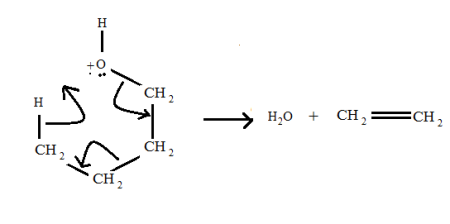A prominent M^+. -18 peak suggests that the compound might be a(n): A. alkane B. alcohol C. ether...

Question:

A prominent M{eq}^+ {/eq}. -18 peak suggests that the compound might be a(n):

A. alkane

B. alcohol

C. ether

D. ketone

E. primary amine

Mass Spectrometry

When a sample of very less concentration is added in the mass spectrometer, it will undergo ionization followed by acceleration.

The ions get deflected inside the chamber under the influence of the magnetic field.

It is thus finally detected and different peaks of mass spectra is observed.

A prominent {eq}M^{+.} -18 {/eq} peak which is observed for alcohol.

Reason:

it can be seen in the given equation that when R-OH gets protonated, a water molecule is released.

The mass of {eq}H_{2}O {/eq} is 18, therefore,{eq}\left [ M - 18 \right ] {/eq} peak is observed in mass spectrometry due to loss of water molecule.